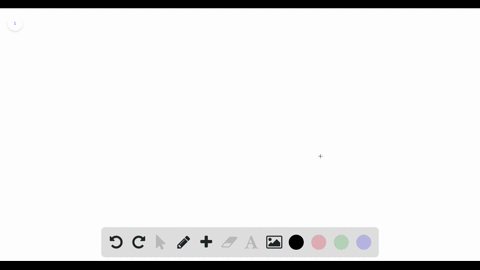Sign up for our free STEM online summer camps starting June 1st!View Summer Courses### $\mathrm{A} 1000 \mathrm{kg}$ boat is traveling a…

02:20
AH
Carnegie Mellon University

Need more help? Fill out this quick form to get professional live tutoring.

Get live tutoring
Problem 32

A block is pushed across a floor by a constant force that is applied at downward angle $\theta($ Fig. $6-19) .$ Figure $6-36$ gives the acceleration magnitude $a$ versus a range of values for the coefficient of
kinetic friction $\mu_{k}$ between block and floor: $a_{1}=3.0 \mathrm{m} / \mathrm{s}^{2}, \mu_{k 2}=$
$0.20,$ and $\mu_{k 3}=0.40 .$ What is the value of $\theta ?$

$\theta=60^{0}$

## Discussion

You must be signed in to discuss.